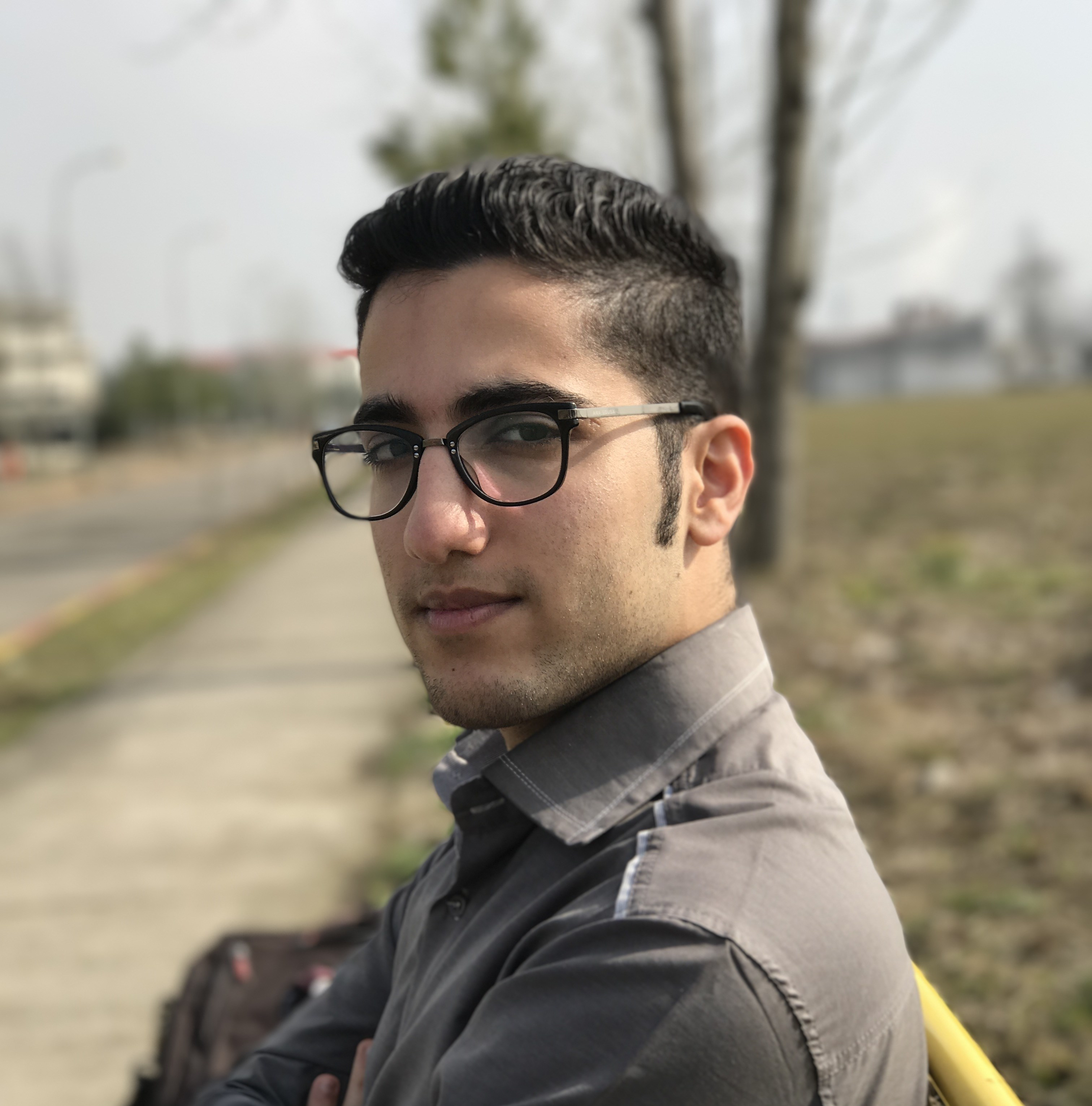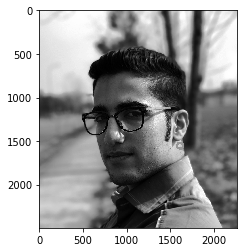# Face Detection, Face Landmarks and dlibNikan Feb 10, 2020 · 4 mins read# Face Detection, Face Landmarks and dlib

This post consists of:

1. dlib is a powerful library in image processing. For detecting face landmarks there are built-in methods in cv2 and dlib
1. Study the codes in linked here and write a program to calculate face landmarks via offline approach and demonstrates them (link1, link2, link3)
1. OpenCV
2. dlib
2. Compare them based on speed and accuracy (use subjective measurement for accuracy)

## 1 dlib is a powerful library in image processing. For detecting face landmarks there are built-in methods in cv2 and dlib

1. Study the codes in linked here and write a program to calculate face landmarks via offline approach and demonstrates them (link1, link2, link3)
1. OpenCV
2. dlib
2. Compare them based on speed and accuracy (use subjective measurement for accuracy)

### 1.A Calculate Face Landmarks

1. OpenCV
2. dlib
Note: I had a few problems with installing dlib so this code has been copied from results I have achieved in Google Colab.
import numpy as np
import cv2
import dlib
import imutils
import matplotlib.pyplot as plt
import time
%matplotlib inline


#### 1.A.a OpenCV

face_cascade = cv2.CascadeClassifier(cv2.data.haarcascades+'haarcascade_frontalface_alt.xml')
image = cv2.imread('IMG.JPG', 0)
image = cv2.equalizeHist(image)
for (x,y,w,h) in faces:
center = (x + w//2, y + h//2)
image = cv2.ellipse(image, center, (w//2, h//2), 0, 0, 360, (255, 0, 0), 4)
plt.imshow(image, cmap='gray')#### 1.A.B dlib

# download data
!wget http://dlib.net/files/shape_predictor_68_face_landmarks.dat.bz2
!bzip2 -dk shape_predictor_68_face_landmarks.dat.bz2

detector = dlib.get_frontal_face_detector()
predictor = dlib.shape_predictor("shape_predictor_68_face_landmarks.dat")
image = cv2.imread('IMG.JPG', 0)
faces = detector(image)
for face in faces:
x1 = face.left()
y1 = face.top()
x2 = face.right()
y2 = face.bottom()
cv2.rectangle(image, (x1, y1), (x2, y2), (0, 255, 0), 3)

landmarks = predictor(image, face)

for n in range(0, 68):
x = landmarks.part(n).x
y = landmarks.part(n).y
cv2.circle(image, (x, y), 4, (255, 0, 0), 4)
plt.imshow(image, cmap='gray')
plt.show()#### 1.B Compare dlib and cv2

Time:

So based on the values we can say that dlib is pure success as only cv2 trained model could not find the face and just marked a small area beneath the ear. Actually, dlib almost found perfect rectangle for face and landmarks are fit on eyes, nose, lip, etc which is enough reason to say that dlib is better. About time, even though the result of dlib is much better, it achieved it approximately 3.57X faster.